# Excel Templates for Manning Equation/Uniform Open Channel Flow Calculations

The use of Excel spreadsheet formulas is a convenient way to make Manning equation calculations for uniform open channel flow. (For background information see "Introduction to the Manning Equation for Open Channel Flow Calculations.") After you’ve set up Excel templates with the appropriate formulas typed in, you can simply type in the values for your known parameters and the Excel spreadsheet formulas will calculate the unknowns that you’re interested in. The next sections of this article have example downloadable Excel templates for several different types of Manning Equation calculations. Each one is presented first with the formulas shown and then with an example completed set of calculations.

## Volumetric Flow Rate and Average Velocity for a Rectangular Channel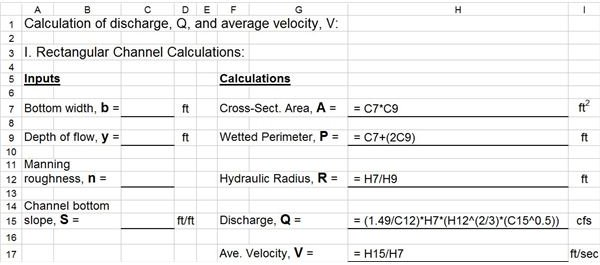The Excel template shown at the left has the formulas to calculate cross-sectional area, wetted perimeter, hydraulic radius, volumetric flow rate (discharge), and average velocity for a rectangular open channel with specified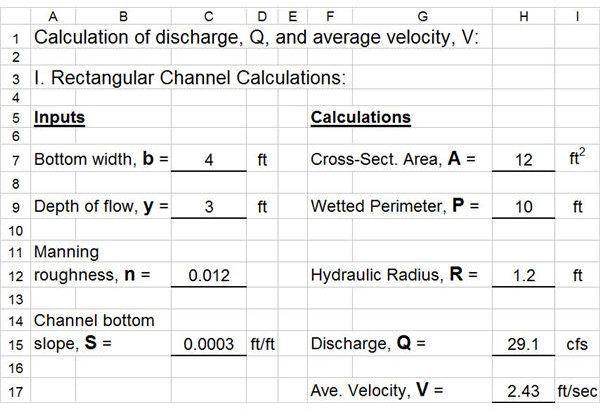bottom width, depth of flow, Manning roughness coefficient, and channel bottom slope. (Click on the image in order to get a larger version that you can read better.) If everything is entered in the Excel rows and columns as shown, then the Excel spreadsheet formulas shown in column H will calculate the parameters, A, P, R, Q, and V using the given values of b, y, n, and S, as shown. The Excel printout at the right shows the results with b = 4 ft, y = 3 ft, n = 0.012, and S = 0.0003. Download this Excel Spreadsheet.

## Volumetric Flow Rate and Average Velocity for a Trapezoidal Channel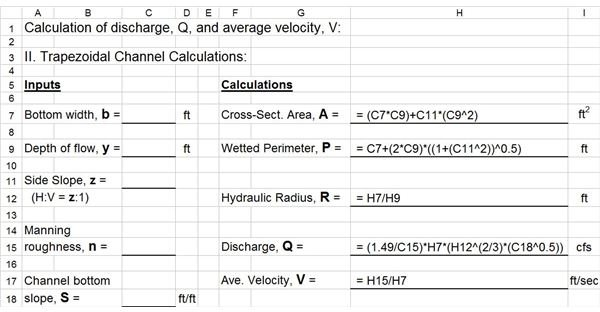This Excel template has the formulas to make calculations similar to those in the previous section, except for a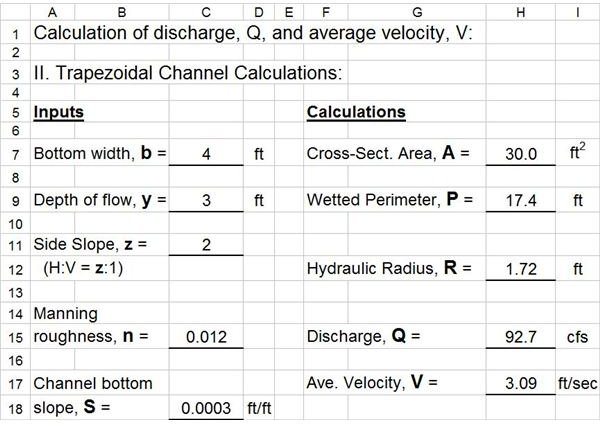trapezoidal cross-section instead of rectangular. The only changes in these Excel spreadsheet formulas from those for the rectangular channel are the formulas for calculating cross-sectional area and wetted perimeter. Once those two parameters are calculated the formulas for hydraulic radius, volumetric flow rate, and average velocity are the same. The Excel printout at the right shows the trapezoidal channel results with b = 4 ft, y = 3 ft, z = 2, n = 0.012, and S = 0.0003. Download this Excel Spreadsheet.

## Slope Needed for Specified Velocity in a Trapezoidal Channel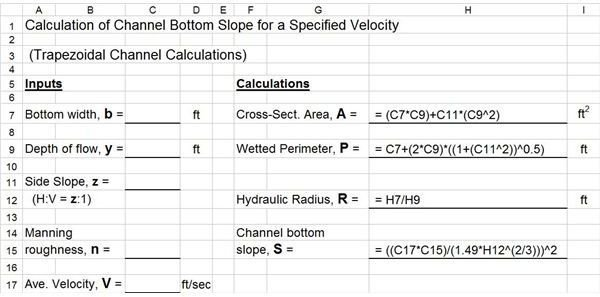This Excel template is set up to calculate the channel bottom slope needed to provide a specified velocity in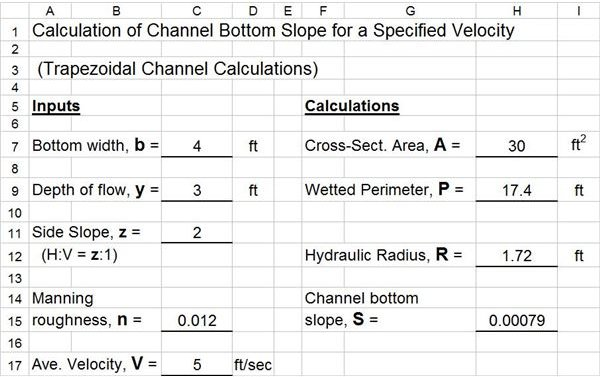a given trapezoidal open channel, flowing at a specified depth. In this case the inputs needed are the channel bottom width, b, the channel side slope, z, the Manning roughness coefficient, n, the depth of flow, y, and the average velocity, V. The Excel spreadsheet formulas are shown in the image at the left and the calculation results are shown in the image at the right for the input values shown. Download this Excel Spreadsheet.

## Experimental Determination of Manning Roughness Coefficient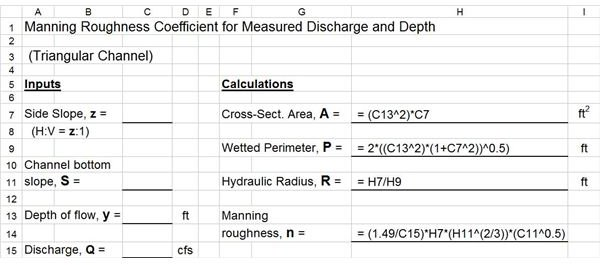The spreadsheet shown at the left will calculate the Manning roughness coefficient, based on measured values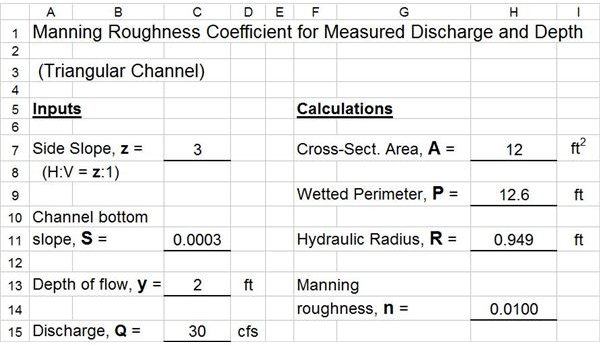of flow depth and volumetric flow rate, for a given triangular channel. The inputs needed are the channel side slope, the channel bottom slope, the depth of flow, and the volumetric flow rate. The Excel spreadsheet formulas shown will then calculate the cross-sectional area of flow, the wetted perimeter, the hydraulic radius, and the Manning roughness coefficient. The image at the right shows the calculated results for a triangular channel with side slope of z = 3, bottom slope of S= 0.0003, flow depth of y = 2 ft, volumetric flow rate of Q = 30 cfs. The resulting experimentally determined Manning roughness coefficient is 0.0100. Download this Excel Spreadsheet.

## Summary

These four example Excel templates illustrate some of the Manning equation calculations for uniform open channel flow that can be done using Excel spreadsheet formulas. Many other similar Excel templates can be set up for other channel shapes or combinations of inputs and unknowns.

## This post is part of the series: Uniform Open Channel Flow and the Manning Equation

The Manning equation is widely used for uniform open channel flow calculations with natural or man made channels. The Manning equation is used to relate parameters like river discharge and water flow velocity to hydraulic radius, and open channel slope, size, shape, and Manning roughness.